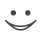Get these flashcards, study & pass exams. For free! Even on iPhone/Android!

Good Measurement (7 Cards)

What do researchers do when they measure a variable?
They observe and record its values.
What is a categorical variable?
This type of variable is sometimes called "nominal" as in name.  The values are categories that do not have a numerical meaning. Examples are: male / female, urban / suburban / rural.
What is a quantitative variable?
A quantitative variable has a numerical scale in which the numbers have value than can be described as more or less. Examples are height and weight.
What are three types of quantitative scales?
Ordinal, interval, and ratio.
What is an ordinal scale?
The measures of a quantitative variable are ordinal when we know the rank order. For an ordinal scale, we only know the order. The distances between levels are unknown. We know that one level is  higher than another level, but not by how much. Star ratings for hotels is an example of a ordinal scale.
What is an interval scale?
An interval scale has the properties of an ordinal scale, but the numbers on an interval scale also have equal distances or intervals between them. There is no true zero on an interval scale which limits what we can say about values coming from the scale. Temperature in degrees Celsius is an interval scale. We can say that the interval between 5 and 10 degrees and 15 and 20 degrees is the same. We cannot say that 0 degrees is "no temperature."
What is an ratio scale?
The ratio scale has the ordinal and interval properties of order and equal intervals. The value of 0 on the scale means there is no quality. Income is an example of a ratio scale. Higher numbers are greater income; intervals are equal all along the scale; and 0 means no income.Flashcard set info:
Author: CoboCards-User
Main topic: Psychology
Topic: Psychology Education
Published: 15.03.2014
Tags: Measurement Scales

Card tags:
All cards (7)
no tagsReport abuse